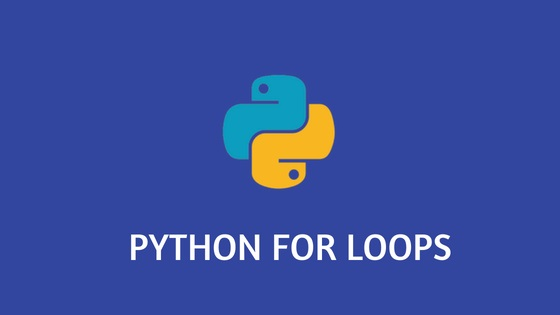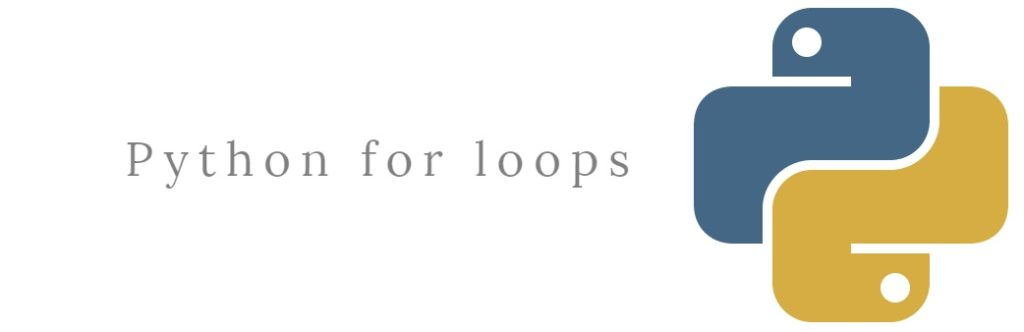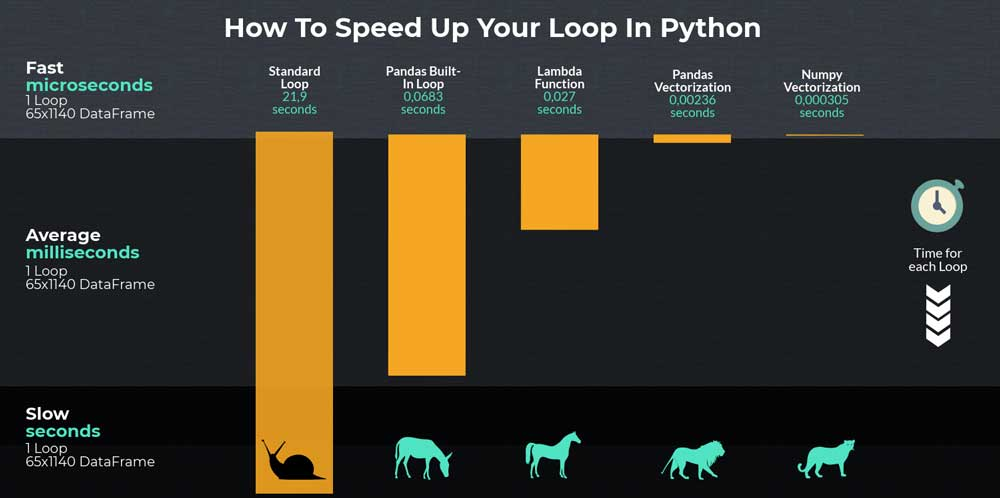# blog posts# What is the whole Python programming language? The for loop for in Python is often called Loop, For is a keyword used as a loop in all programming languages, including Python.

Records show that in the past, programming languages ​​used iterative loops in various structures, which we will briefly discuss.

As you know, the for loop in Python is one of the most important types of control commands. The for loop is used for control commands. This open loop is used for various sequences.

### Ring limited to a specific number

The iteration loop in Python is more of a simple loop with a certain numerical range that has a numeric value to start and end. The exact format of the for loop depends on the programming language, but normally most of the mentality we have about the for loop is as follows:

Here the body of the loop will be repeated 10 times. The variable i will be set to 1 in the first step and to 2 in the second step, and will continue to the end of the loop. This type of iteration loop is used for BASIC, Algol and Pascal languages.### The loop repeats three phrases

There is another form of the popular for iteration loop in the C programming language that has three parts:

• Initialization
• The second statement considers a condition for the end of the loop
• The third phrase identifies the action performed at the end of each iteration

The general form of the command is as follows:

Technical Note: In the C programming language, the i ++ operation means that a value is added to the variable i. This operation is approximately equal to i + = 1 in Python.
This loop is interpreted as follows:

• Variable i is initially set to 1.
• The loop continues until the variable i has not yet reached 10.
• At the end of each loop, a value is added to the variable i (i ++).

Rings with the possibilities of three expressions are very popular because in these three expressions, any kind of state can be established and this circle can be managed in different ways, and in general, it can be said that this type of circle is of flexibility. It has a lot of high simplicity. These types of loops are also more common in C ++, Java, PHP and Perl.

### Collection-based repetition loop

These types of loops are mostly used on a set of objects instead of specifying the values ​​of the numerical range:

Each time the loop is repeated, the variable i assigns an object of the set in order. The type of these rings is more abstract and general. Perl and php languages ​​support these types of loops, but use Foreach instead of the For keyword. If you are also interested in hacking, getting to know Python hacking is a good article.

### for as a repeating loop in PythonOf all the rings mentioned above, Python follows only the last ring, the ring based on a set of objects. Implementing an iteration loop in Python is very diverse and you will always have a new way to implement an iteration loop in Python.
You will find out more about the iteration loop in Python and the structure of the for loop in Python, but for now we will only get a little more familiar with it in the early examples.

## The structure of for as a iterative loop in Python is as follows:

Here <iterable> is in the iteration loop in Python as a set of objects or, for example, a list of objects. Statements are executed in the body of the loop for each item of <iterable>. The variable var allocates an iterable value each time the loop is repeated.
Here is an example of a repetition loop in Python:

In this loop the iteration in Python <iterable> is the same as list a and the variable <var> is i. Each time the loop of variable i is repeated, it continuously assigns some of the items in list a, then the print () function displays the values ​​’foo’, ‘bar’ and ‘baz’, respectively. For repeat loop like this is a Python method used to process items in the iteration step.
But what exactly can be an iterable loop loop in Python? Before we look at most of the loops, it’s a good idea to delve deeper into what is really meant by iterable loop loops in Python. In the following article, we suggest you to know Python programming language better .

### Iterable

In the iteration loop in Python iterable is an object that can be used in iteration. The term is used as follows:

• Adjective: An object can be described as a duplicate.
• Name: An object is specified as a duplicate.If an object is iterable, it can be called in the iter () function in Python, which returns something called an iterator.
Each of the objects in the example above is an iterable and returns the iterator or iterator type when returned to the iter () function.

#### On the other hand, in the following example, these objects returned to the iter function are not iterable:

All the data types you have come across so far that are collection type are iterable. These types of data that are iterable include data such as string, list, tuple, dict, set and fixed lists.

But this data is not the only type that you can use in the iteration loop in Python. There are many objects designed and defined in Python that you can use as iterable in the Python loop.

#### for example:

An open file in the iteration loop in Python can be used as an iterabe. You can use an open file as iterable and read objects from it and use it in your iterations after you have learned enough about the iteration loop in Python.

Virtually any object in Python can be repeat as an iterator in the iteration loop in Python. Even user-defined objects can be design to be used instead of iterators or iterators (in this article you will learn how we can benefit from object-oriented programming in Python).

### Iterators in the for loop for in Python

You now have a good idea of ​​what data types are known as iterable, and you understand how to use the iter () function to get an iterator. Now that we have access to an iterator, what can we do with it?In the iteration loop in Python, an iterator is essentially a value generator that assigns sequential values ​​to the corresponding iterable object.
The constructor function next () in the iteration loop in Python is use to assign the next value to the iterator.

Here is an example using the same list above:

In this example, a is an iterable list and itr is an iterator that was first converted to an iterator by the iter () function. Each next command (itr) returns the next value in this list in order.

Notice how a repeater maintains its inner state. The repeater realizes that the values ​​are predetermined, so when you call the next () function in the iteration loop in Python, the repeater realizes that it must return the next value.